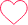# Find the Greatest of the Three Numbers​ in Python

## Find the Greatest of the Three Numbers

Given three integer inputs the objective is to find the largest among them. Therefore we write a code to Find the Greatest of the Three Numbers in Python.

```Example
Input : 20 30 10
Output : 30
```## Find the Greatest of the Three Numbers in Python Language

Given three integers as inputs the objective is to find the greatest among them. In order to do so we check and compare the three integer inputs with each other and which ever is the greatest we print that number. Here are some methods to solve the above problem.

• Method 1: Using if-else Statements
• Method 2: Using Nested if-else Statements
• Method 3: Using Ternary Operator

We’ll discuss the above mentioned methods in-depth in the upcoming sections.

### Method 1: Using if-else Statements

In this method we use if-else statements to find the Largest Number among the three integer inputs.

### Working

For integer inputs number1, number2 and number3

• Initialize the required variables.
• Using if-else check if the numbers are greater than max and change max if true.
• Print max variable.

Let’s implement the working in Python Language.

### Python Code

Run
```num1, num2, num3 = 10 , 30 , 20
max = 0
if num1 >= num2 and num1 >= num3:
print(num1)
elif num2 >= num1 and num2 >= num3:
print(num2)
else:
print(num3)
```

```30
```

### Method 2: Using Nested if-else Statements

In this method we use if-else statements within one another to find the Largest Number among the three integer inputs.

### Working

For integer inputs number1, number2 and number3

• Initialize the required variables.
• Using nested if-else check if the numbers are greater than max and change max if true.
• Print max variable.

Let’s implement the working in Python Language.

### Python Code

Run
```num1, num2, num3 = 10 , 350 , 550
max = 0
if num1 >= num2:
if num1 >= num3:
print(num1)
elif num2 >= num1:
if num2 >= num3:
print(num2)
else :
print(num3)
```

```30
```

### Method 3: Using Ternary Operator

In this method we use Ternary operator to compare and find the Largest Number among the three integer inputs.

### Working

For integer inputs number1, number2 and number3

• Initialize the required variables.
• Using Ternary Operator check if the numbers are greater than max and change max if true.
• Print max variable.

Let’s implement the working in Python Language.

### Python Code

Run
```num1, num2, num3 = 10 , 30 , 20
max = num1 if num1>num2 else num2
max = num3 if num3>max else max
print(max)
```

```30
```

### Related Banners

Get PrepInsta Prime & get Access to all 200+ courses offered by PrepInsta in One Subscription

### 12 comments on “Find the Greatest of the Three Numbers​ in Python”

•realrishiiscool Gaming

x = int(input(“Enter first number”))
y = int(input(“Enter second number”))
z = int(input(“Enter third number”))
if (x>y>z):
print(x,”is the biggest”)
elif (y>z>x):
print(y,”is the biggest”)
else:
print(z,”is the biggest”)0
•Raghvendra Singh Shekhawat

N1 = int(input(“Enter the first number : “))
N2 = int(input(“Enter the second number : “))
N3 = int(input(“Enter the third number : “))

####### METHOD – 1

greatest = max(N1,N2,N3)
print(f”{greatest} is the greatest of the three numbers”)

###### METHOD – 2

if N1 > N2 and N1 > N3:
print(N1)
elif N2 > N1 and N2 > N3:
print(N2)
elif N3 > N1 and N3 > N2:
print(N3)
else:
print(“All the numbers are same”)0
•prajwalkamde264

num1 = int(input(“Enter num1 : “))
num2 = int(input(“Enter num2 : “))
num3 = int(input(“Enter num3 : “))

if num1 > num2 and num1 > num3:
print(f”Greatest number is {num1}”)
elif num2 > num1 and num2 > num3:
print(f”Greatest number is {num2}”)
elif num3 > num1 and num3 > num2:
print(f”Greatest number is {num3}”)
else:
print(“All numbers are equal”)0
•Aniket

a = int(input())
b = int(input())
c = int(input())

if a>b:
if a>c:
print(f”{a} is greatest”)
else:
print(f”{c} is greatest”)
else:
if b>c:
print(f”{b} is greatest”)
else:
print(f”{c} is greatest”)3
•Bibhudutta

a=int(input())
b=int(input())
c=int(input())

if a>b and a>c:
print(a,’ is greatest of all ‘)
elif b>a and b>c:
print(b,’ is greatest of all’)
else:
print(c,’ is greatest of all’)0
•Shubham

first = int(input(“Enter first number:”))
second = int(input(“Enter second number:”))
third = int(input(“Enter third number:”))
if first > second > third:
print(“First is Greatest”)
elif second > third > first:
print(“Second is Greatest”)
else:
print(“Third is Greatest”)0
•rohitnamdev876

n1 = int(input(“Enter the number 1 : “))
n2 = int(input(“Enter the number 2 : “))
n3 = int(input(“Enter the number 3 : “))
if n1 < n2 :
if n2 <n3:
print(f"{n3} is Grater")
else:
print(f"{n2} is Grater")
else:
print(f"{n1} is grater ")0
•M

num1 = int(input(“enter the number:”))
num2 = int(input(“enter the number:”))
num3 = int(input(“enter the number:”))
if (num1 > num2) and (num1 > num3):
print(str(num1) + ” the number is greater”)
elif (num2 > num1) and (num2 > num3):
print(str(num2) + ” the number is greater”)
else:
print(str(num3) + ” the number is greater”)0
•a= int(input(“enter number a :”))
b= int(input(“enter number b:”))
c= int(input(“enter number c :”))
print (maximum(a,b,c))

def maxi(a,b,c):
list=[a,b,c]
return max(list)

a=2
b=6
c=8
print(maxi(a,b,c))0
•SOUMIK

we can do it with function calling method also.
def Gratestnumber(num1,num2,num3):
if (num1>num2) and (num1>num3):
print(“num1 is gratest”)
elif (num2>num1) and (num2>num3):
print(“num2 is gratest”)
else:
print(“num3 is gratest”)
Gratestnumber(num1,num2,num3)1
•pavan

x=int(input(“Enter the first number:”))
y=int(input(“Enter the second number:”))
z=int(input(“Enter the third number:”))
if (x>y and x>z):
print(“X is greater”)
elif (y>z and y>x):
print(“Y is greater”)
else:
print (“Z is greater”)0
•Sai

first = int(input(“Enter first number:”))
second = int(input(“Enter second number:”))
third = int(input(“Enter third number:”))
greatest = max(first,second,third)
print(“Greatest number is:”,greatest)0